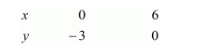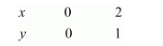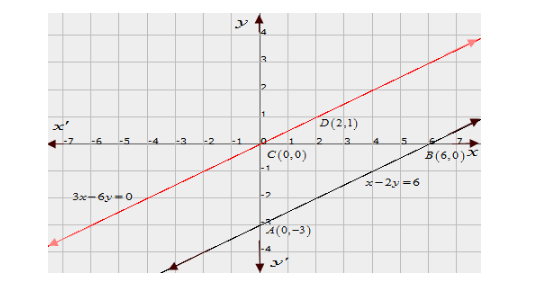# Show graphically that each one of the following systems of equations is in-consistent (i.e. has no solution) :`
Question:

Show graphically that each one of the following systems of equations is in-consistent (i.e. has no solution) :

$x-2 y=6$

$3 x-6 y=0$

Solution:

The given equations are

$x-2 y=6$$.(i)$

$3 x-6 y=0$..(ii)

Putting $x=0$ in equation $(i)$, we get:

$\Rightarrow 0-2 y=6$

$\Rightarrow y=-3$

$\Rightarrow x=0, \quad y=-3$

Putting $y=0$ in equation $(i)$ we get:

$\Rightarrow x-2 \times 0=6$

$\Rightarrow x=6$

$\Rightarrow x=6, \quad y=0$

Use the following table to draw the graph.The graph of $(i)$ can be obtained by plotting the two points $A(0,-3), B(6,0)$.

Graph of the equation.... (ii):

$3 x-6 y=0$ .. (ii)

Putting $x=0$ in equation (ii) we get:

$\Rightarrow 3 \times 0-6 y=0$

$\Rightarrow y=0$

$\Rightarrow x=0, \quad y=0$

Putting $y=1$ in equation $(i i)$, we get:

$\Rightarrow 3 x-6 \times 1=0$

$\Rightarrow x=2$

$\Rightarrow x=2, \quad y=1$

Use the following table to draw the graph.Draw the graph by plotting the two points $C(0,0), D(2,1)$ from table.Here the two lines are parallel and so there is no point in common

Hence the given system of equations has no solution.